Ch 1. Particle General Motion Multimedia Engineering Dynamics Position,Vel & Accel. Accel. varyw/ Time Accel. Constant Rect. Coordinates Norm/Tang. Coordinates Polar Coordinates RelativeMotion
 Chapter - Particle - 1. General Motion 2. Force & Accel. 3. Energy 4. Momentum - Rigid Body - 5. General Motion 6. Force & Accel. 7. Energy 8. Momentum 9. 3-D Motion 10. Vibrations Appendix Basic Math Units Basic Equations Sections Search eBooks Dynamics Fluids Math Mechanics Statics Thermodynamics Author(s): Kurt Gramoll ©Kurt GramollDYNAMICS - CASE STUDY SOLUTION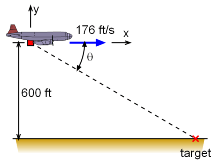Problem Diagram To find the angle θ, the horizontal distance, x needs to be known. However, the package is moving in both the x and y direction. First, start with a diagram at the instant the package is released as shown at the left. Position the origin of the Cartesian coordinate system at the point where the package is released, with the positive y axis pointing up. Resolve the acceleration into rectangular components,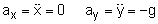and integrate these equations over time to get velocity, and then again to get position. In addition to the two differential equations, initial conditions (i.e. boundary conditions) for time, position and velocity are needed to solve the equations. The initial conditions are:      Initial Position:  xo = 0, yo = 0      Initial Velocity:  vxo, = 176 ft/s, vyo = 0      Initial Time:  to = 0 Velocity and Position (Horz. Direction) Integrating the acceleration equation with respect to time gives,      dvx = ax dt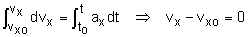vx = vxo = 176 ft/s Since the x-direction velocity is constant, the horizontal velocity does not change while the package is falling. The horizontal position can be determined by integrating vx = over time,      dx = vx dt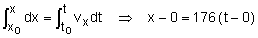x = 176 t The difficulty with this result, is the time for the package to hit the ground is not known. Thus, vertical direction now needed to determine t. Velocity and Position (Vertical Direction) Just like for the horizontal direction, the vertical velocity and position can be determined by integrating the acceleration equation,      dvy = ay dt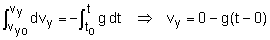vy = -gt Integrating vy over time will give the y position,      dy = vy dt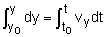The vertical distance to the ground is known, 600 ft,     600 - 0 = -g/2 (t - 0)2      t = 6.10 s Angle Now that the time to impact is know, the horizontal distance can be calculated,      x = 176 (6.10) = 1,074 ft Trigonometry can be used to find the angle θ:      θ = tan-1 (600/1074) = 29.2o The falling package exemplifies the characteristics of projectile motion.

Practice Homework and Test problems now available in the 'Eng Dynamics' mobile app
Includes over 400 problems with complete detailed solutions.
Available now at the Google Play Store and Apple App Store.